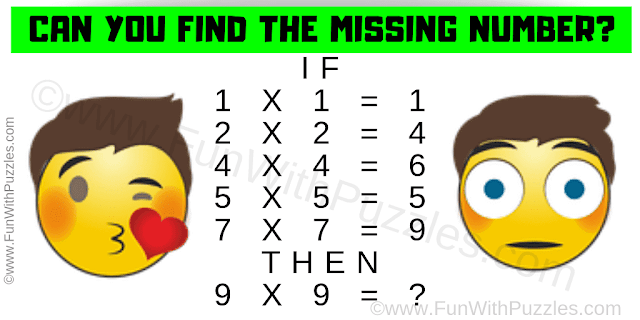It is a very interesting logical math question for students in which your challenge is to find the missing number which will replace the question mark. In this Logical Math Question, you are shown one number equation. Decipher the logical pattern used in these equations to find the value of the last equation. Find the missing number which will replace the question mark in the last equation.Can you solve this Logical Math Question?
Answer of this "Logical Math Question", can be viewed by clicking on the answer button.